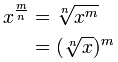Exponents ICSE Class-8th Concise Selina Power Solutions Chapter-2. We provide step by step Solutions of Exercise / lesson-2 Exponents ICSE Class-8th Concise Selina Mathematics. Our Solutions contain all type Questions with Exe-2 A and Exe-2 B,  to develop skill and confidence. Visit official Website for detail information about ICSE Board Class-8.

## Exponents ICSE Class-8th Concise Selina Power Solutions Chapter-2

–: Select Topics :–

Exe-2 A,

Exe-2 B,

Laws of Exponents / Power

Law Example
x1 = x 61 = 6
x0 = 1 70 = 1
x-1 = 1/x 4-1 = 1/4
xmxn = xm+n x2x3 = x2+3 = x5
xm/xn = xm-n x6/x2 = x6-2 = x4
(xm)n = xmn (x2)3 = x2×3 = x6
(xy)n = xnyn (xy)3 = x3y3
(x/y)n = xn/yn (x/y)2 = x2 / y2
x-n = 1/xn x-3 = 1/x3
And the law about Fractional Exponents:### Exercise – 2 A Exponents ICSE Class-8th Concise Selina Power Solutions

#### Question 1 :-

Evaluate:#### Question 2 :-

If 1125 = 3m x 5n; find m and n.

1125 = 32 x 53

The factor of 1125 are 3 x 3 x 5 x 5 x 5

 3 1125 3 375 5 125 5 25 5 5 1

∴ 1125 = 3 x 3 x 5 x 5 x 5

Now comparing, 32 x 53 = 3m x 5n

∴ m = 2, n = 3

#### Question 3 :-

Find x, if 9 × 3x = (27)2x-3

9 x 3x = (27) 2x-3

Since, bases are same, compare them,

x + 2 = 6x – 9

6x – x = 9 + 2

⇒ 5x = 11

⇒ x = 11/2

⇒ x = 215

### Exe -2 B  Exponents / Power ICSE Class-8th Concise selina

#### Question 1 :-

Compute:#### Question 2 :-

Simplify:
(i)
(ii) [(64)-2]-3 ÷ [{(-8)2}3]2
(iii) (2-3 – 2-4) (2-3 + 2-4)(ii)

(iii)

#### Question 3 :-

Evaluate:
(i) (5)0
(ii) 80+40+20

(iii) (8+4+2)0

(iv) 4X0

(v) (4x)0

(vi) [(103)0)0]5

(vii) (7X0)2

(viii) 90+9-19-2+ 91/2 91/2

(i) (5)0

(5)0 =1

( a0 =1)

(ii)

(iii)

(iv)

(v)

(vi)

(vii)

(viii)

90+9-19-291/2 − 91/2

Simplify:

(i)

(ii)

(iii)

(iv)

(v)

(vi)

(vii)

(viii)

(i)

(ii)

(iii)

(iv)

(v)

(vi)

(vii)

(viii)

#### Question 5 :-

Simplify:
(xa+b)a-b.(xb+c)b-c.(xc+a)c-a

(xa+b)a-b.(xb+c)b-c.(xc+a)c-a

(xa+b)(a-b).(xb+c)(b-c).(xc+a)(c-a)

=xa²-b². xb²-c².. xc²-a².

= x+b²- +

=x0

=1

Simplify:
(i)
(ii)

(i)

(ii)

#### Question 7 :-

(i) (a-2)-2. (ab)-3
(ii) (xny-m)× (x3y-2)-n
(iii)
(iv)
(v) (a-2b)1/2 × (ab-3)1/3
(vi) (xy)m-n . (yz)n-1 . (zx)1-m

(i)

(ii)

(iii)

(iv)

(v)

(vi)

Show that:

Evaluate:

Evaluate:

#### Question 11 :-

(m + n)-1 (m-1 + n-1) = (mn)-1

Hence Proved.

Prove that:

(i)

(ii)

(i)

(ii)

#### Question 13 :-

Find the values of n, when:
(i) 12-5 × 122n+1 = 1213 ÷ 127
(ii)

(i)

(ii)

#### Question 14 :-

Simplify:

(i)

(ii)

(i)

(ii)

— End of Exponents ICSE Class-8th Concise Solutions :–

Thanks